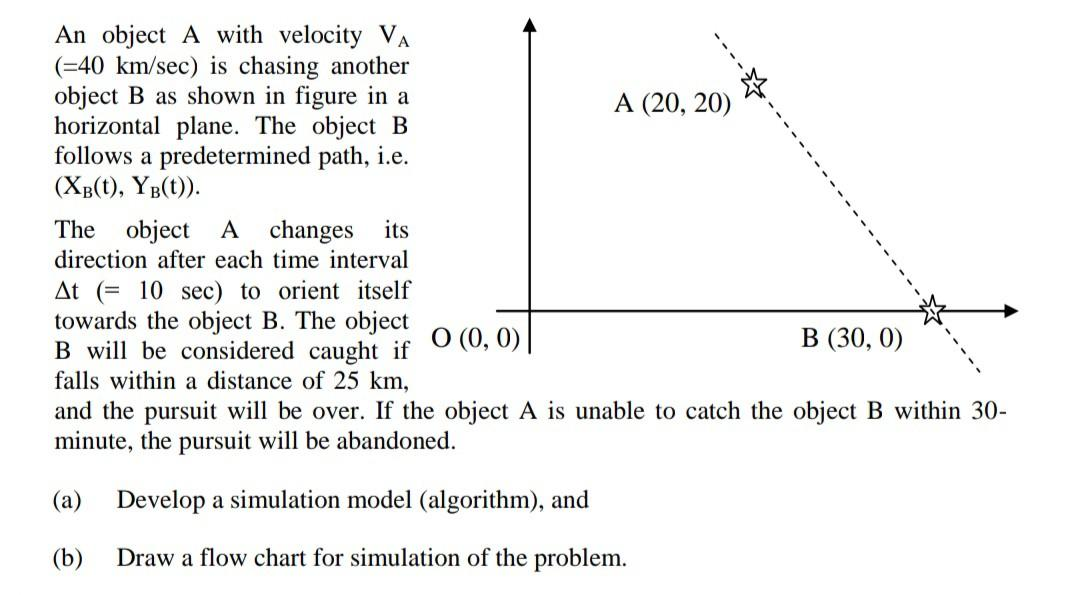# An object A with velocity VA (=40 km/sec) is chasing another object B as shown in figure in a horizontal plane. The object B follows a predetermined path, i.e. (XB(t), YB(t)).

Question-AnswerCategory: Modelling & SimulationAn object A with velocity VA (=40 km/sec) is chasing another object B as shown in figure in a horizontal plane. The object B follows a predetermined path, i.e. (XB(t), YB(t)).

An object A with velocity VA
(=40 km/sec) is chasing another
object B as shown in figure in a
horizontal plane. The object B
follows a predetermined path, i.e.
(XB(t), YB(t)).